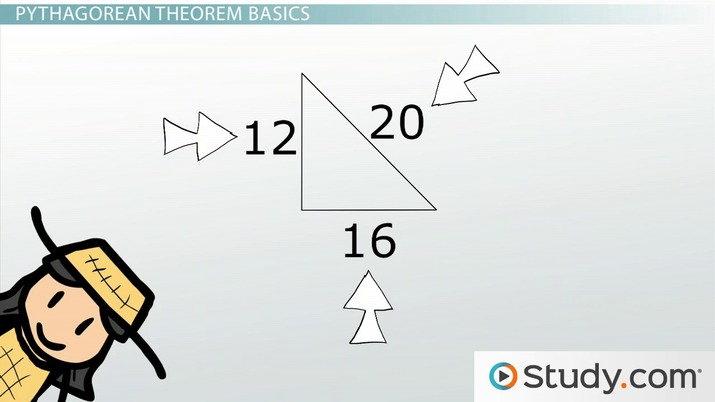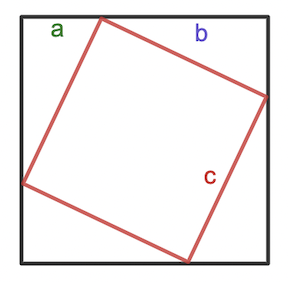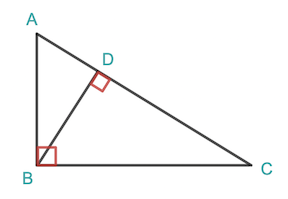# Pythagorean Theorem: Overview, Formula, & Examples

Lisa Gilbert, DaQuita Hester
• Author
Lisa Gilbert

Lisa received a Bachelor's of Business Administration from the University of Georgia. Upon graduation, she worked as a Human Resources Manager for 7 years, managing all aspects of human resource management including writing and delivering training for employees. For the last 15 years, Lisa has reviewed a vast array of curriculum and taught and tutored all subjects to homeschooled students in all grades pre-K through early college.

• Instructor
DaQuita Hester

DaQuita has taught high school mathematics for six years and has a master's degree in secondary mathematics education.

Learn what the Pythagorean theorem is. Discover how to prove and use the Pythagorean theorem with examples, and identify how this theorem is used in real life. Updated: 02/06/2022

Show

## What Is the Pythagorean Theorem?

The definition of the Pythagorean theorem, or Pythagoras' theorem, states that the sum of the squares of the two legs of a right triangle is equal to the sum of the square of the hypotenuse. The hypotenuse is the longest side of a right triangle and is always opposite the right angle. This theorem is used in determining the length of the sides of a right triangle. The formula for the Pythagorean theorem is {eq}a^2 + b^2 = c^2 {/eq}, where a and b are the legs and c is the hypotenuse.

### Pythagoras

Pythagoras was a fifth century BCE Greek philosopher and mathematician who is credited with several scientific and mathematical discoveries, most notably the Pythagorean theorem. Although the Pythagorean theorem was popularized by Pythagoras, other ancient mathematicians in ancient civilizations such as Babylon and China had previously formulated the idea. Pythagoras was the first to prove its validity for all right triangles.An error occurred trying to load this video.

Try refreshing the page, or contact customer support.

Coming up next: How to Identify Similar Triangles

### You're on a roll. Keep up the good work!

Replay
Your next lesson will play in 10 seconds
• 0:06 The Theorem and Its Purpose
• 1:49 Pythagorean Theorem Basics
• 4:22 Pythagorean Theorem in…
• 6:56 Lesson Summary
Save Save

Want to watch this again later?

Timeline
Autoplay
Autoplay
Speed Speed

## Pythagoras Theorem Proof

The Pythagorean theorem can be proven in several different ways. To prove the theorem algebraically, begin with a diagram of a tilted square inside another square.By tilting the inner square so that its corners touch the sides of the outer square, four congruent right triangles are formed, each with sides a and b and a hypotenuse c. The sides of the larger square are equal to a + b, and the sides of the inner square are equal to c. The area of the larger square can be determined in two ways: {eq}A = (a + b)^2 {/eq} OR the sum of the areas of the four triangles and the inner square. Since both methods produce the same result, the two methods are equal. So, mathematically,

{eq}(a + b)^2 = 4(ab/2) + c^2\\\ a^2 + 2ab + b^2 = 2ab + c^2\\\ a^2 + b^2 = c^2 {/eq}

Another way to prove Pythagoras' theorem is by using the similar triangle method. Start by constructing a right triangle. Then, from the vertex of the right angle, draw a line perpendicular to the hypotenuse, as indicated in the diagram.angle A = angle A common angle angle ADB = angle ABC right angles triangle ADB is similar to triangle ABC angle-angle similarity

For triangles BDC and ABC:

 angle C = angle C common angle angle CDB = angle CBA right angles triangle BDC is similar to triangle ABC angle-angle similarity

Thus, all three triangles are similar, so their sides are proportionate.

 AB/AC = AD/AB, so AB2 = AD ⋅ AC cross multiplication BC/AC = CD/BC, so BC2 = CD ⋅ AC cross multiplication AB2 + BC2 = (AD ⋅ AC) + (CD ⋅ AC) add both equations together AB2 + BC2 = AC(AD + CD) factoring AB2 + BC2 = AC(AC) substitution AB2 + BC2 = AC2 multiplication

Pythagoras' theorem is proven!

Another mathematician, Euclid, proved the theorem in yet another way by constructing squares from each of the sides of the right triangle. Euclid's method is often referred to as the Windmill proof because its shape resembles that of a windmill.## Using the Pythagorean Theorem Formula

Since the Pythagorean theorem has been proven valid by many different methods, the formula {eq}a^2 + b^2 = c^2 {/eq} can be reliably used to find the missing side length of a right triangle. The length of a hypotenuse can be calculated using the formula when the other two side lengths are given, or the length of one leg can be calculated if only a leg length and hypotenuse are given. For example, use Pythagoras' formula to find the hypotenuse when the lengths of the other two sides are 3 and 4. To find the hypotenuse, substitute 3 and 4 for a and b, then solve for c.

{eq}3^2 + 4^2 = c^2\\\ 9 + 16 = c^2\\\ 25 = c^2\\\ 5 = c {/eq}

Note: When practicing how to do the Pythagorean theorem, be sure to square the a and b terms before adding them together.

To unlock this lesson you must be a Study.com Member.

#### How do you find C in the Pythagorean theorem?

To find c, or the hypotenuse, first find the squares of a and b. Then, find the square root of the sum of those squares.

#### What is Pythagoras' theorem used for?

Pythagoras' theorem is used for finding the length of a missing side of a right triangle. It can be used to find a leg length or the hypotenuse length.

#### What is the formula for the Pythagorean Theorem?

The formula for the Pythagorean theorem is the sum of the squares of the two legs equal to the square of the hypotenuse. Mathematically, the formula is {eq}a^2 + b^2 = c^2 {/eq}, where a and b are the legs and c is the hypotenuse.

### Register to view this lesson

Are you a student or a teacher?